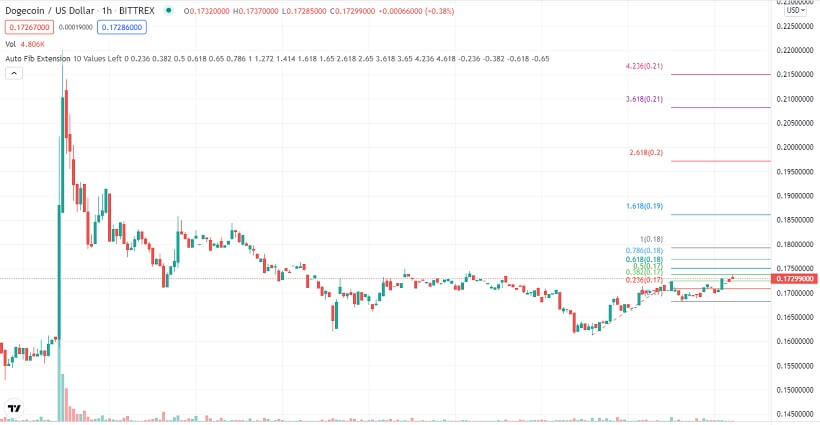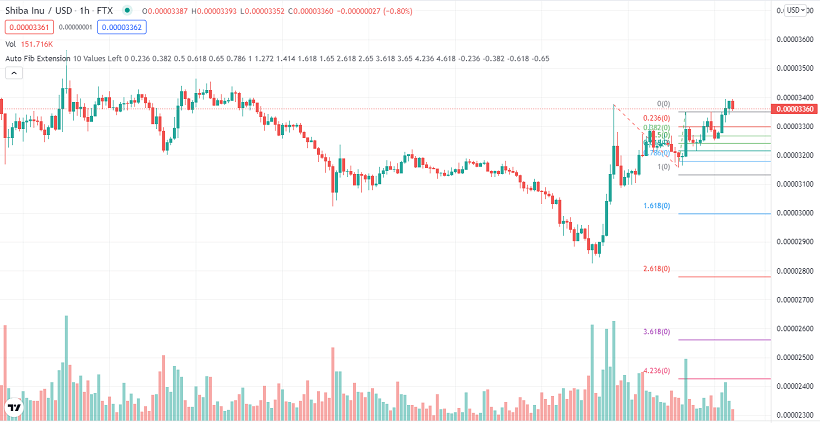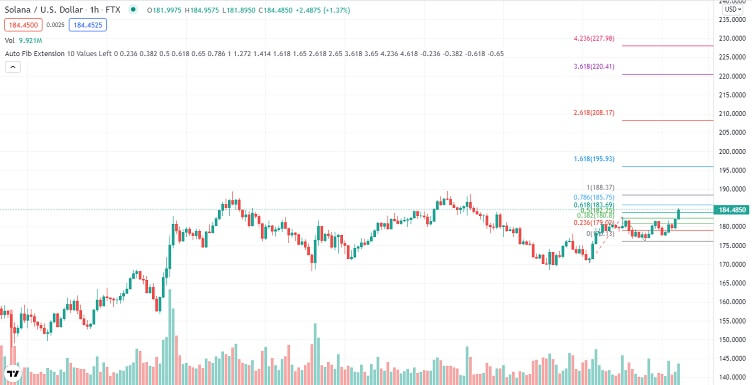# Dogecoin, Shiba Inu, and Solana Technical Analysis – 22nd Dec

## Key resistance levels are in focus as upside momentum rises in the crypto market

Last Updated December 22nd 2021
• Dogecoin bulls take control, but they need to first overcome the 78.6% Fibonacci resistance at \$0.1731.
• Shiba Inu bulls show weakness just below the 78.6% Fibonacci resistance at \$0.00003405.
• Solana gaining upside momentum as bulls push SOL through the 23.6% Fibonacci resistance at \$181.41

## Dogecoin

Dogecoin was range-bound for the better part of Tuesday. By the end of the day, it was up by about 2.3%.

Dogecoin started Tuesday trading at \$0.1670 on the 38.2% Fibonacci support after a correction in late Monday trading.

Dogecoin traded along the 38.2% Fibonacci for about 3-hours before bulls took control and pushed it through the 50.0% Fibonacci resistance at \$0.1687.

Upside momentum was quite strong, and just before daybreak, Dogecoin had pushed through the 61.8% Fibonacci resistance at \$0.1705.

However, Dogecoin bulls lost momentum at the 61.8% Fibonacci resistance at \$0.1705. What followed was range-bound trading along with this resistance level until early afternoon.

At this point, bears took control after bulls struggled, but failed, to push Dogecoin through the 61.8% Fib.

Bearish momentum was short-lived, though, and within 2-hours, bears hit strong support at \$0.1687 on the 50.0% Fib.

Bulls attempted to take control for the second time in the day, albeit with low volumes. Dogecoin kept edging higher, and by late evening, had pushed through the 61.8% Fibonacci resistance at \$0.1705.

However, just an hour before the end of the day, bears took control and pushed Dogecoin back through the 61.8% Fib.

Dogecoin started trading Wednesday bearish, continuing the correction that started in late Tuesday trading.

Just two hours into the day, bulls took control and pushed Dogecoin back through the 61.8% Fibonacci resistance.

When going to press, Dogecoin was testing the 78.6% Fibonacci resistance at \$0.1731.### A glance at the day ahead

The key levels to watch are the 78.6% Fibonacci resistance at \$0.1731 and the 61.8% Fibonacci, now support at \$0.1687.

If bulls take control and push Dogecoin through the 78.6% Fibonacci resistance at 0.1731 the next key level to watch would be the multi-week resistance at \$0.1765.

If the multi-week resistance breaks, prices above \$0.19 could be seen within the day.

However, if the 61.8% Fibonacci support at \$0.1687 is broken, the next level to watch would be the 50.0% Fibonacci support at \$0.1688. If the 50.0% Fibonacci support is broken, prices below \$0.165 could be tested within the day.

On the other hand, if volumes drop, Dogecoin could trade between the 78.6% Fibonacci resistance at \$0.1731 and the 61.8% Fibonacci support at \$0.1687.

### A glance at the technicals

Key resistance: 78.6% Fibonacci at \$0.1731

Key support: 61.8% Fibonacci at \$0.1687

## Shiba Inu

Shiba Inu was strongly bullish in Tuesday trading. By the end of the day, it was up by 6%.

Shiba Inu started trading Tuesday, bouncing off the 38.2% Fibonacci support at \$0.00003105.

Momentum was quite strong, and by daybreak, Shiba Inu had blasted through the 50.0% Fibonacci resistance at \$0.00003194 and was trading at the 61.8% Fibonacci resistance at \$0.00003281.

However, the 61.8% Fibonacci resistance proved too strong for bulls, and a correction followed.

Shiba Inu was in a correction until early afternoon when it hit the 50.0% Fibonacci, now support at \$0.00003194.

Bears briefly managed to push Shiba Inu through the 50.0% Fibonacci support but failed. What followed was a rally that saw Shiba Inu blast through the 61.8% Fibonacci resistance with ease.

However, momentum dropped, and a slight correction back through the 61.8% Fib followed.

The correction did not last, though, and in the early evening, bulls took control. What followed was a rally back through the 61.8% Fibonacci all through the evening.

In the last hour of the day, Shiba Inu had a correction back to the 61.8% Fibonacci.

Shiba Inu started Wednesday trading in a correction, continuing the price action from late Tuesday trading. However, by the third hour of the day, bulls were in control. What followed was a rally back through the 61.8% Fibonacci resistance.

When going to press, Shiba Inu was close to testing the 78.6% Fibonacci resistance at \$0.00003405.### A glance at the day ahead

The key levels to watch are the 61.8% Fibonacci now support at \$0.00003281 and the 78.6% Fibonacci resistance at \$0.00003405.

If bulls take control and push Shiba Inu through the 78.6% Fibonacci resistance at \$0.00003405, the next key level to watch would be the 50.0% Fibonacci resistance at \$0.00003304.

If the 50.0% Fibonacci resistance is broken, monthly resistance at \$0.00003563 would come into focus. If this resistance is broken, prices above \$0.00003601 could be possible in the day.

However, if the 61.8% Fibonacci support at \$0.00003281 breaks; the next key level to watch would be the 50.0% Fibonacci support at \$0.00003194. If the 50.0% Fib is broken, prices below \$0.00003146 could be seen in the day.

On the other hand, if volumes drop, Shiba Inu could trade between the 78.2% Fibonacci resistance at \$0.00003405 and the 61.8% Fibonacci support at \$0.00003281.

### A glance at the technicals

Key resistance: 78.6% Fibonacci at \$0.00003405

Key support: 61.8% Fibonacci at \$0.00003281

## Solana

Solana was bullish for the better part of Tuesday. By the end of the day, it was up by 4%.

Solana started Tuesday trading in the red, a continuation of the selloff that had begun in late Monday trading.

However, bears lost momentum three hours into the day, and Solana pushed back through the 50.0% Fibonacci support at \$172.84.

Upside momentum accelerated, and by noon, Solana had broken the 38.2% Fibonacci resistance at \$176.69 and was trading at the 23.6% Fibonacci resistance at \$181.41.

The 23.6% Fibonacci resistance proved too strong for bulls, and a correction followed. By early evening, Solana was trading at the 38.2% Fibonacci, now support at \$176.69.

Solana bounced off the 38.2% Fibonacci, and just two hours to the end of the day hit the 23.6% Fibonacci resistance at \$181.41.

Bulls failed to push Solana through the 23.6% Fibonacci support for the second time in the day, leading to a correction in the last two hours of the day.

Solana started bullish after bears lacked the momentum to push it back to the 38.2% Fibonacci support in late Tuesday trading.

Just before daybreak, Solana retested the 23.6% Fib resistance but again failed to push through it.

This led to a correction, and Solana was still in a correction when going to press.### A glance at the day ahead

The key levels to watch are the 23.6% Fibonacci resistance at \$181.41 and the 38.2% Fibonacci support at \$179.69.

If bulls take control and push Solana through the 23.6% Fibonacci resistance, the next key level to watch would be the weekly high of \$189.44.

If Solana bulls manage to push it through the weekly high of \$189.44, prices above \$200 could be seen in the day.

However, if bears take control and push Solana through the 38.2% Fibonacci support at \$176.69, the next key level to watch would be 50.0% Fibonacci support at \$172.84.

If the 50.0% Fibonacci support is broken, prices below \$170 could be hit within the day.

On the other hand, if volumes drop, Solana could trade between the 23.6% Fibonacci resistance at \$181.41 and the 38.2% Fibonacci support at \$179.69.

### A glance at the technicals

Key resistance: 23.6% Fibonacci at \$181.41

Key support: 38.2% Fibonacci at \$179.69